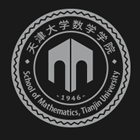﻿An Eigenwise Parallel Augmented Subspace Method for Eigenvalue Problems-天津大学数学学院

# An Eigenwise Parallel Augmented Subspace Method for Eigenvalue Problems

2019-08-26 10:44

A type of parallel augmented subspace scheme for eigenvalue problems is proposed by using coarse space in the multigrid method. With the help of coarse space in multigrid method, solving the eigenvalue problem in the finest space is decomposed into solving the standard linear boundary value problems and very low dimensional eigenvalue problems. The computational efficiency can be improved since there is no direct eigenvalue solving in the finest space and the multigrid method can act as the solver for the deduced linear boundary value equations. Furthermore, for different eigenvalues, the corresponding boundary value problem and low dimensional eigenvalue problem can be solved in the parallel way since they are independent of each other and there exists no data exchanging. This property means that we do not need to do the orthogonalization in the highest dimensional spaces. This is the main aim of this paper since avoiding orthogonalization can improve the scalability of the proposed numerical method.### 联系我们